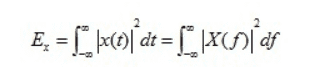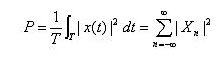# Parseval's theorm

## Homework Statement

In parseval's theorm, what is type of x(t)?? I mean.. is this voltage wave? or power wave?

## Homework Equations## The Attempt at a Solution

#### Attachments

•Delta2

Delta2
Homework Helper
Gold Member
Mathematically, ##x(t)## can be any function ##x:\mathbb{R}\rightarrow\mathbb{C}## that is any complex valued function over the real line (or as a sub case any real valued function over the real line ##x:\mathbb{R}\rightarrow\mathbb{R}##) for which its continuous fourier transform ##X(f)## exists . So it can be a function representing the (complex) voltage between two nodes of a circuit or the power between two nodes of a circuit.

However the usual interpretation of this theorem in signal analysis is that the two sides of the equation are just two different ways of computing the total energy of a signal ##x(t)## (a voltage or a current signal).

•Jiho
you mentioned it doesn't matter that signal x(t) represents voltage or power. But I can't understand if x(t) represent power,this equation doesn't make sense as I think. In my opinion, How can power^2 be just power?? Unit of dimension is not same as I know.

#### Attachments

Delta2
Homework Helper
Gold Member
Yes I said that ##x(t)## can be any function for which the fourier transform exists. However I also said that the usual interpretation is that of energy (the integrals in both sides represent energy) in the case ##x(t)## is a voltage or current signal. If ##x(t)## is a power signal then we cant give that usual interpretation of energy to this theorem.

•Jiho